## Lesson 9.1 Understanding the concept of relations

### 9.101 Introduction

• Relationships between elements of sets occur in many contexts.
• We deal with relationships in a daily basis:
• A relationship between a person and a relative.
• A relationship between an employee and their salary.
• A relationship between a business and its telephone number.
• A relationship between a computer language and a valid statement in this language will often arise in computer science.
• In maths, we study relationships like:
• the relation between a positive integer and one it divides.
• relation between a real number and one that is larger than it.
• relation between a business and its telephone number.
• relation between a computer language and a valid statement
• relation between a real number x and the value f(x) where f is a function, and so on.
• Relations in maths
• We can define relationship between elements of 2 sets
• We can also define the relationship between 2 elements of a set.

### 9.103 Definition of a relation: relation versus function

• Relation
• A relation can be defined between elements of set A and elements of another set B.
• Can also be defined between elements of the same set.
• We use the letter $R$ to refer to relation.
• Let $A$ and $B$ be two sets.
• Let $R$ be a relation linking elements of set $A$ to elements of set $B$.
• Let $x \in A$ and $y \in B$
• We say that $x$ is related to $y$ with respect to relation $R$ and we write $x \ R \ y$
• A relation can also be between elements of the same set.
• A relation is a link between two elements of a set
• For example
• A person x is a SON OF' a person y.
• SON OF is a relation that links x to y
• Usually use the letter $R$ to refer to a relation:
• In this case $R$ = 'SON OF'
• If $x$ is SON OF $y$ we write $x \ R \ y$
• If $y$ is NOT a SON OF $x$ we write $y \ \not R \ x$
• A relation can be defined as a link or connection between elements of set A to set B.
• Example
• A is the set of students in a Comp Science class
• $A = \{Sofia, Samir, Sarah\}$
• B is the courses the department offers
• $B = \{Maths, Java, Databases, Art\}$
• Let $R$ be a relation linking students in set $A$ to classes they are enrolled in: A student is related to the course if the student is enrolled in the course.
• Examples:
• Sofia is enrolled in Math and Java
• Samir is enrolled in Java and Databases
• Sarah is enrolled in Math and Art
• Sofia is not enrolled in Art
• Notations:
• Sofia $R$ Maths
• Sofia $R$ Java
• Samir $R$ Java
• Samir $R$ Databases
• Sarah $R$ Maths
• Sarah $R$ Art
• Sofia $\not R$ Art
• Cartesian product
• Let $A$ and $B$ be 2 sets.
• The Cartesian product A x B is defined by a set of pairs (x, y) such that $x \in A$ and $y \in B$
• $A \ x \ B = \{(x, y): x \in A \text{ and } y \in B \}$
• For example:
• $A = \{ a_1, a_2 \}$ and $B = \{b_1, b_2, b_3\}$
• $A \ x \ B = \{(a_1, b_1), (a_1, b_2), (a_1, b_3), (a_2, b_1), (a_2, b_2), (a_2, b_3)\}$
• Definition of relation
• Let $A$ and $B$ be two sets.
• A binary relation from A to B is a subset of a Cartesian product $A \ x \ B$
• $R \subseteq A \ x \ B$ means $R$ is a set of ordered pairs of the form (x, y) where $x \in A$ and $y \in B$.
• $(x, y) \in R$ means $x \ R \ y$ (x is related to y)
• For example:
• $A = \{a, b, c\}$ and $B = \{1, 2, 3\}$
• The following is a relation defined from A to B:
• $R = \{(a, 1), (b, 2), (c, 3)\}$
• This means that: $a\ R \ 1$, $b \ R \ 2$ and $c \ R \ 3$
• Relations on a set
• When A = B
• A relation R on the set A is a relation from A to A
• $R \subseteq A \ x \ A$
• We will be studying relations of this type.
• Example
• A = {1, 2, 3, 4}
• Let R be a relation on the set A:
• $x, y \in A$, $x \ R \ y$ if and only if $x < y$
• We have 1 R 2, 1 R 3, 1 R 4, 2 R 3, 2 R4, 3 R 4
• $R = \{(1,2), (1,3),(1,4), (2, 3), (2, 4), (3, 4)\}$

### 9.105 Matrix and graph representatins of a relation

• Relations using matrices
• Given a relation R from a set A to set B.
• List the elements of sets A and B in a particular order
• Let $n_a = |A|$ and $n_b = |B|$
• The matrix of R is an $n_a \ \text{x} \ n_b$ matrix.
• $M_r = [m_{ij}] n_a \ x \ n_b$
• In matrix store a 1 if $(a_i, b_j) \in R$ otherwise, 0
• Example 1
• Let A = {Sofia, Samir, Sarah}
• Let B = {CS100, CS101, CS102, CS103}
• Consider the relation of who is enrolled in which class
• R = { (a,b) | person a is enrolled in course b }
CS100 CS101 CS102
Sofia x x
Samir x x
Sarah x x

$M_r = \begin{bmatrix} 1 & 1 & 0 \\ 0 & 1 & 1 \\ 1 & 0 & 1 \end{bmatrix}$

• Example 2
• Let A = { 1, 2, 3, 4, 5 }
• Consider a relation: $< (x, y) \in R \text{ if and only if } x < y$
• Every element is not related to itself (hence the diagonal 0s).

$M_r = \begin{bmatrix} 0 & 1 & 1 & 1 & 1 \\ 0 & 0 & 1 & 1 & 1 \\ 0 & 0 & 0 & 1 & 1 \\ 0 & 0 & 0 & 0 & 1 \\ 0 & 0 & 0 & 0 & 0 \\ \end{bmatrix}$

• Example 3
• Let A = { 1, 2, 3, 4, 5 }
• Consider a relation : $\leq (x, y) \in R$ if and only if $x \leq y$
• Note the diagonal is all 1s.

$M_r = \begin{bmatrix} 1 & 1 & 1 & 1 & 1 \\ 0 & 1 & 1 & 1 & 1 \\ 0 & 0 & 1 & 1 & 1 \\ 0 & 0 & 0 & 1 & 1 \\ 0 & 0 & 0 & 0 & 1 \\ \end{bmatrix}$

• Combining relations
• Union
• The Union of 2 relations is a new set that contains all of the pairs of elements that are in at least one of the two relations.
• The union is written as $R \ U \ S$ or "R or S".
• $R \ U \ S$ = { $(a, b): (a, b) \in R$ or $(a, b) \in S$ }
• Intersection
• The intersection of 2 relations is a new set that contains all of the pairs that are in both sets.
• The intersection is written as $R \cap S$ or "R and S"
• $R \cap S$ = { $(a, b): (a, b) \in R$ and $(a, b) \in S$ }
• Combining relations: via Boolean operators
• Let $M_{R} = \begin{bmatrix}1 & 0 & 1 \\ 1 & 0 & 0 \\ 0 & 1 & 0 \end{bmatrix} \\ M_{s} = \begin{bmatrix} 1 & 0 & 1 \\ 0 & 1 & 1 \\ 1 & 0 & 0 \end{bmatrix}$
• Join $M_{R \cup S} = M_R \vee M_S = \begin{bmatrix}1 & 0 & 1 \\ 1 & 1 & 1 \\ 1 & 1 & 0\end{bmatrix}$
• Meet $M_{R \cap S} = M_R \land M_S = \begin{bmatrix} 1 & 0 & 1 \\ 0 & 0 & 0 \\ 0 & 0 & 0 \end{bmatrix}$
• Representing relations using directed graphs
• When a relation is defined on a set, we can represent with a digraph.
• Building the digraph
• First, the elements of A are written down,
• When $(a, b) \in R$ arrows are drawn from a to b.
• Example 1
• A = { 1, 2,3 ,4}
• Let R be relation on A defined as follows:
• R = { $(x, y)$ | x divides y}
• R can be represented by this digraph
•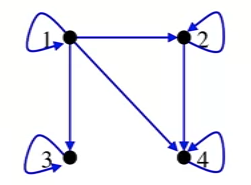• Each value divides itself, hence the loop at each vertex.
• One divides all other elements, so it has a link to each elements.
• 2 only divides into 4
• So on...
• Example 2
• Let A = { 1, 2, 3, 4, 5 }
• Consider relation: $\leq (x, y) \in R$ if and only if $x \leq y$
•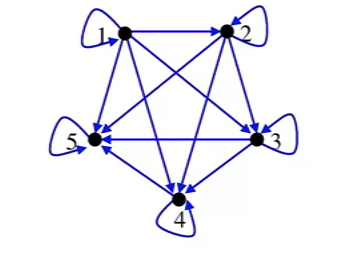• Each element is equal to itself.
• One is less than or equal to all elements.
• 5 is greater than all elements except itself.
• So on.

### 9.107 The properties of a relation: reflexive, symmetric and anti-symmetric

• Reflexivity
• A relation R in a set S is said to be reflexive if and only if $x \ R \ x$, $\forall x \in S$
• Or, for all x in the set, if the pairs (x, x) is in the relation, then it's reflexive.
• Example 1 (reflexive example)
• Let R be a relation of elements in Z:
• $R = \{ (a, b) \in Z^2 | a \leq b \}$
• For all x elements of Z, we have $x \leq x$, hence $x \ R \ x$
• This implies that R is reflexive.
• Example 2 (non-reflexive)
• $R = \{ (a, b) \in Z^2 | a \lt b \}$
• Digraph of reflexive relation
• Every element will have a loop.
• In this example, S = {1, 2, 3, 4} and R is a relation of elements S $R = \{ (a, b) \in S^2 | a \leq b \}$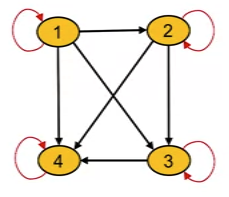• Matrix of reflexive relation
• Same example as above.
• Note that all the values in the diagonal are 1.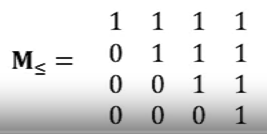• Definition of Symmetry
• A relation is said to be symmetric if and only if:
• $\forall a, b \in S$, if $a \ R \ b$ then $b \ R \ a$.
• Proof: let $a, b \in Z$ with $a \ R \ b$:
• a mod 2 = b mod 2
• b mod 2 = a mod 2
• b R a
• R is symmetric
• Diagram of a symmetric relation
• Example
• Let S = {1, 2, 3, 4} and R be relation of elements in S
• R = { (a,b) \in S^2 | a mod 2 = b mod 2 }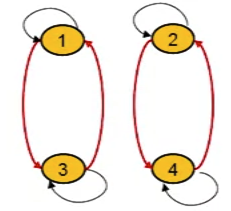• Matrix of symmetric relation
• Example
• Let S = {1, 2, 3, 4} and let R be relation of elements in S
• R = { (a, b) \in S^2 | a mod 2 = b mod 2 }
• $M_R = \begin{bmatrix}1 & 0 & 1 & 0 \\ 0 & 1 & 0 & 1 \\ 1 & 0 & 1 & 0 \\ 0 & 1 & 0 & 1\end{bmatrix}$
• Definition of anti-symmetric
• A relation R on a set S is said to be anti-symmetric if and only if $\forall a, b \in S$, if $a \ R \ b$ and $b \ R \ a$ then $a = b$
• In other words, no 2 diff elements are related both ways.
• Examples
• Let R be a relation on elements in Z:
• $R = \{ (a, b) \in Z^2 | a \leq b \}$
• Let $a, b \in Z$, $a \ R \ b$ and $b \ R \ a$
• $a \leq b$ and $b \leq a$
• $a = b$
• R is anti-symmetric
• Digraph of anti-symmetric relation
• Digraph contains no parallel edges between any 2 different vertices.
• Example
• Let S = {1, 2, 3, 4} and R be relation on elements in S
• $R = \{ (a, b) \in S^2 | \text{ a divides b } \}$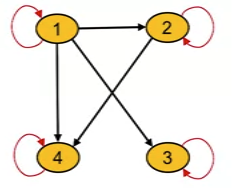• Matrix of an anti-symmetric relation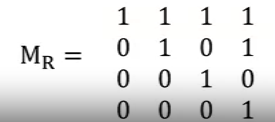• Exercise
• Let R be the relation defined by the Matrix M_r
• $M_r = \begin{bmatrix} 0 & 0 & 1 \\ 1 & 1 & 1 \\ 0 & 0 & 1 \end{bmatrix}$
• Is R reflexive? Symmetric? Anti-symmetric?
• Clearly R is not reflexive: $m_{1,1} = 0$
• It is not symmetric because $m_{2,1}=1, m_{1, 2}=0$
• However, it is anti-symmetric.

### 9.109 Relation properties: transitivity

• Definition of transitivty
• A relation $R$ on a set S is called transitive if and only if:
• $\forall a, b, c \in S$, if ($a \ R \ b$ and $b \ R \ c$) then $a \ R \ c$
• Examples of transitive relations
• $R = \{ (x , y) \in N^{2} | x \leq y \}$
• It is transitive as $\forall x, y, z \in \mathbf{N}$ if $x \leq y$ and $y \leq z$ then $x \leq z$
• R = { (a, b) | a is an ancestor of b }
• It is transitive because if a is an acestory of b and b is an ancestor of c, then a is an ancestor of c.
• Example of non-transitive relations
• R = { (2, 3), (3, 2), (2, 2) }
• It is not transitive because 3 R 2 and 2 R 3 but 3 \not R 3
•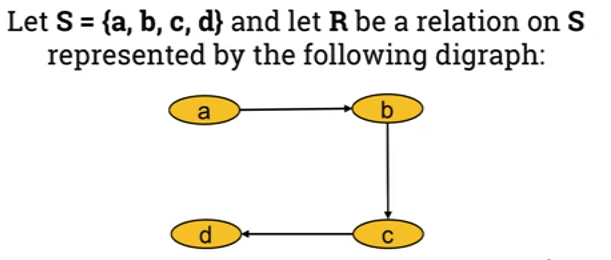• Not transitive as:
• $a \ R \ c$ and $b \ R \ c$, however $a \ \not R \ c$
• Also: $b \ R \ c$ and $c \ R \ d$ however $b \ \not R \ d$
• What edges need to be added to make it transitive?
•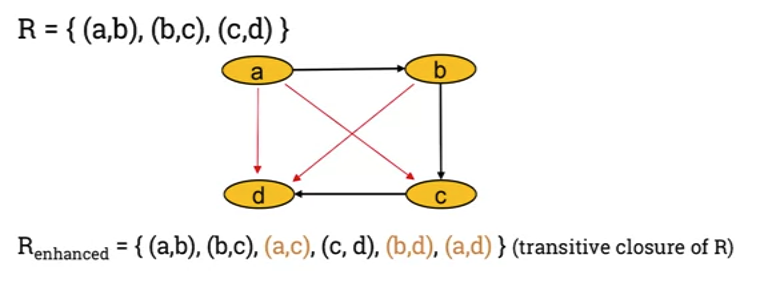• The result enhanced relation is called the "transitive closure of the original relation"